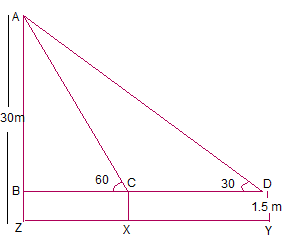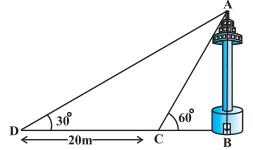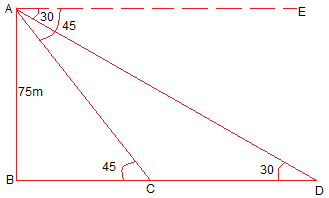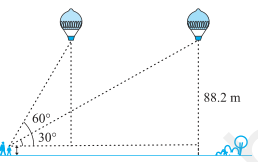# NCERT Solutions for Class 10th: Ch 9 Some Applications of Trigonometry Maths

#### NCERT Solutions for Class 10th: Ch 9 Some Applications of Trigonometry Maths

Excercise: 9.1

Page No: 2031. A circus artist is climbing a 20 m long rope, which is tightly stretched and tied from the top of a vertical pole to the ground. Find the height of the pole, if the angle made by the rope with the ground level is 30° (see Fig. 9.11).

Let AB be the vertical pole Ac be 20 m  long rope tied to point C.
In  right ΔABC,
sin 30° = AB/AC
⇒ 1/2 = AB/20
⇒ AB = 20/2
⇒ AB = 10
The height of the pole is 10 m.

2. A tree breaks due to storm and the broken part bends so that the top of the tree touches the ground making an angle 30° with it. The distance between the foot of the tree to the point where the top touches the ground is 8 m. Find the height of the tree.

∴ Total height of the tree = AB+AC
In  right ΔABC,
cos 30° = BC/AC
⇒ √3/2 = 8/AC
⇒ AC = 16/√3
Also,
tan 30° = AB/BC
⇒ 1/√3 = AB/8
⇒ AB = 8/√3
Total height of the tree = AB+AC = 16/√3 + 8/√3 = 24/√3

3. A contractor plans to install two slides for the children to play in a park. For the children below the age of 5 years, she prefers to have a slide whose top is at a height of 1.5 m, and is inclined at an angle of 30° to the ground, whereas for elder children, she wants to have a steep slide at a height of 3 m, and inclined at an angle of 60° to the ground. What should be the length of the slide in each case?

There are two slides of height 1.5 m and 3 m. (Given)
Let AB is 1.5 m and PQ be 3 m slides.
ABC is the slide inclined at 30° with length AC and PQR is the slide inclined at
60° with length PR.
A/q,
In  right ΔABC,
sin 30° = AB/AC
⇒ 1/2 = 1.5/AC
⇒ AC = 3m
also,
In  right ΔPQR,
sin 60° = PQ/PR
⇒ √3/2 = 3/PR
⇒ PR = 2√3 m
Hence, length of the slides are 3 m and 2√3 m respectively.

Page No: 204

4. The angle of elevation of the top of a tower from a point on the ground, which is 30 m away from the foot of the tower, is 30°. Find the height of the tower.

Let AB be the height of the tower and C is the point elevation which is 30 m away from the foot of the tower.
A/q,
In  right ΔABC,
tan 30° = AB/BC
⇒ 1/√3 = AB/30
⇒ AB = 10√3
Thus, the height of the tower is 10√3 m.

5. A kite is flying at a height of 60 m above the ground. The string attached to the kite is temporarily tied to a point on the ground. The inclination of the string with the ground is 60°. Find the length of the string, assuming that there is no slack in the string.

Let BC be the height of the kite from the ground,
AC be the inclined length of the string from the ground and A is the point where string of the kite is tied.
A/q,
In  right ΔABC,
sin 60° = BC/AC
⇒ √3/2 = 60/AC
⇒ AC = 40√3 m
Thus, the length of the string from the ground is 40√3 m.

6.  A 1.5 m tall boy is standing at some distance from a 30 m tall building. The angle of elevation from his eyes to the top of the building increases from 30° to 60° as he walks towards the building. Find the distance he walked towards the building.Answer

Let the boy initially standing at point Y with inclination 30° and then he approaches the building to
the point X with inclination 60°.
∴ XY is the distance he walked towards the building.
also, XY = CD.
Height of the building = AZ = 30 m
AB = AZ - BZ = (30 - 1.5) = 28.5 m
A/q,
In  right ΔABD,
tan 30° = AB/BD
⇒ 1/√3 = 28.5/BD
⇒ BD = 28.5√3 m
also,
In  right ΔABC,
tan 60° = AB/BC
⇒ √3 = 28.5/BC
⇒ BC = 28.5/√3 = 28.5√3/3 m
∴ XY = CD = BD - BC = (28.5√3 - 28.5√3/3) = 28.5√3(1-1/3) = 28.5√3 × 2/3 = 57/√3 m.
Thus, the distance boy walked towards the building is 57/√3 m.

7.  From a point on the ground, the angles of elevation of the bottom and the top of a transmission tower fixed at the top of a 20 m high building are 45° and 60° respectively. Find the height of the tower.

Let BC be the 20 m high building.
D is the point on the ground from where the elevation is taken.
Height of transmission tower = AB = AC - BC
A/q,
In  right ΔBCD,
tan 45° = BC/CD
⇒ 1 = 20/CD
⇒ CD = 20 m
also,
In  right ΔACD,
tan 60° = AC/CD
⇒ √3 = AC/20
⇒ AC = 20√3 m
Height of transmission tower = AB = AC - BC = (20√3 - 20) m = 20(√3 - 1) m.

8.  A statue, 1.6 m tall, stands on the top of a pedestal. From a point on the ground, the angle of elevation of the top of the statue is 60° and from the same point the angle of elevation of the top of the pedestal is 45°. Find the height of the pedestal.

Let AB be the height of statue.
D is the point on the ground from where the elevation is taken.
Height of pedestal = BC = AC - AB
A/q,
In  right ΔBCD,
tan 45° = BC/CD
⇒ 1 =  BC/CD
⇒ BC = CD.
also,
In  right ΔACD,
tan 60° = AC/CD
⇒ √3 = AB+BC/CD
⇒ √3CD = 1.6 m + BC
⇒ √3BC = 1.6 m + BC
⇒ √3BC - BC = 1.6 m
⇒ BC(√3-1) = 1.6 m
⇒ BC = 1.6/(√3-1) m
⇒ BC = 0.8(√3+1) m
Thus, the height of the pedestal is 0.8(√3+1) m.

9. The angle of elevation of the top of a building from the foot of the tower is 30° and the angle of elevation of the top of the tower from the foot of the building is 60°. If the tower is 50 m high, find the height of the building.

Let CD be the height of the tower equal to 50 m (Given)
Let AB be the height of the building.
BC be the distance between the foots of the building and the tower.
Elevation is 30° and 60° from the tower and the building respectively.
A/q,
In  right ΔBCD,
tan 60° = CD/BC
⇒ √3 = 50/BC
⇒ BC = 50/√3
also,
In  right ΔABC,
tan 30° = AB/BC
⇒ 1/√3 = AB/BC
⇒ AB = 50/3
Thus, the height of the building is 50/3.

10. Two poles of equal heights are standing opposite each other on either side of the road, which is 80 m wide. From a point between them on the road, the angles of elevation of the top of the poles are 60° and 30°, respectively. Find the height of the poles and the distances of the point from the poles.

Let AB and CD be the poles of equal height.
O is the point between them from where the height of elevation taken.
BD is the distance between the poles.
A/q,
AB = CD,
OB + OD = 80 m
Now,
In  right ΔCDO,
tan 30° = CD/OD
⇒ 1/√3 = CD/OD
⇒ CD = OD/√3 ... (i)
also,
In  right ΔABO,
tan 60° = AB/OB
⇒ √3 = AB/(80-OD)
⇒ AB = √3(80-OD)
AB = CD (Given)
⇒ √3(80-OD) = OD/√3
⇒ 3(80-OD) = OD
⇒ 240 - 3 OD = OD
⇒ 4 OD = 240
⇒ OD = 60
Putting the value of OD in equation (i)
CD = OD/√3 ⇒ CD = 60/√3 ⇒ CD = 20√3 m
also,
OB + OD = 80 m ⇒ OB = (80-60) m = 20 m
Thus, the height of the poles are 20√3 m and distance from the point of elevation are 20 m and 60 m respectively.11.  A TV tower stands vertically on a bank of a canal. From a point on the other bank directly opposite the tower, the angle of elevation of the top of the tower is 60°. From another point 20 m away from this point on the line joining this point to the foot of the tower, the angle of elevation of the top of the tower is 30° (see Fig. 9.12). Find the height of the tower and the width of the canal.

Here, AB is the height of the tower.
CD = 20 m (given)
A/q,
In  right ΔABD,
tan 30° = AB/BD
⇒ 1/√3 = AB/(20+BC)
⇒ AB = (20+BC)/√3 ... (i)
also,
In  right ΔABC,
tan 60° = AB/BC
⇒ √3 = AB/BC
⇒ AB = √3 BC ... (ii)
From eqn (i) and (ii)
AB = √3 BC = (20+BC)/√3
⇒ 3 BC = 20 + BC
⇒ 2 BC = 20 ⇒ BC = 10 m
Putting the value of BC in eqn (ii)
AB = 10√3 m
Thus, the height of the tower 10√3 m and the width of the canal is 10 m.

12.  From the top of a 7 m high building, the angle of elevation of the top of a cable tower is 60° and the angle of depression of its foot is 45°. Determine the height of the tower.

Let AB be the building of height 7 m and EC be the height of tower.
A is the point from where elevation of tower is 60° and the angle of depression of its foot is 45°
EC = DE + CD
also, CD = AB = 7 m.
A/q,
In  right ΔABC,
tan 45° = AB/BC
⇒ 1= 7/BC
⇒ BC = 7 m = AD
also,
⇒ √3 = DE/7
⇒ DE = 7√3 m
Height of the tower = EC =  DE + CD
= (7√3 + 7) m = 7(√3+1) m.

13. As observed from the top of a 75 m high lighthouse from the sea-level, the angles of depression of two ships are 30° and 45°. If one ship is exactly behind the other on the same side of the lighthouse, find the distance between the two ships.Let AB be the lighthouse of height 75 m.
Let C and D be the positions of the ships.
30° and 45° are the angles of depression from the lighthouse.
A/q,
In  right ΔABC,
tan 45° = AB/BC
⇒ 1= 75/BC
⇒ BC = 75 m
also,
In  right ΔABD,
tan 30° = AB/BD
⇒ 1/√3 = 75/BD
⇒ BD = 75√3  m
The distance between the two ships = CD = BD - BC = (75√3 - 75) m = 75(√3-1) m.

Page No: 20514.  A 1.2 m tall girl spots a balloon moving with the wind in a horizontal line at a height of 88.2 m
from the ground. The height of 88.2 m from the ground. The angle of elevation of the balloon from the eyes of the girl at any instant is 60°. After some time, the angle of elevation reduces to 30° (see Fig. 9.13). Find the distance travelled by the balloon during the interval.

Let the initial position of the balloon be A and final position be B.
Height of balloon above the girl height = 88.2 m - 1.2 m = 87 m
Distance travelled by the balloon =
DE = CE - CD
A/q,
In  right ΔBEC,
tan 30° = BE/CE
⇒ 1/√3= 87/CE
⇒ CE = 87√3 m
also,
⇒ √3= 87/CD
⇒ CD = 87/√3 m = 29√3 m
Distance travelled by the balloon =  DE = CE - CD = (87√3 - 29√3) m = 29√3(3 - 1) m = 58√3 m.

15.  A straight highway leads to the foot of a tower. A man standing at the top of the tower observes a car at an angle of depression of 30°, which is approaching the foot of the tower with a uniform speed. Six seconds later, the angle of depression of the car is found to be 60°. Find the time taken by the car to reach the foot of the tower from this point.

Let AB be the tower.
D is the initial and C is the final position of the car respectively.
Angles of depression are measured from A.
BC is the distance from the foot of the tower to the car.
A/q,
In  right ΔABC,
tan 60° = AB/BC
⇒ √3 = AB/BC
⇒ BC = AB/√3 m
also,
In  right ΔABD,
tan 30° = AB/BD
⇒ 1/√3 = AB/(BC + CD)
⇒ AB√3 = BC + CD
⇒ AB√3 = AB/√3 + CD
⇒ CD = AB√3 - AB/√3
⇒ CD = AB(√3 - 1/√3)
⇒ CD = 2AB/√3
Here, distance of BC is half of CD. Thus, the time taken is also half.
Time taken by car to travel distance CD = 6 sec.
Time taken by car to travel BC = 6/2 = 3 sec.

16.  The angles of elevation of the top of a tower from two points at a distance of 4 m and 9 m from the base of the tower and in the same straight line with it are complementary. Prove that the height of the tower is 6 m.

Let AB be the tower.
C and D be the two points with distance 4 m and 9 m from the base respectively.
A/q,
In  right ΔABC,
tan x = AB/BC
⇒ tan = AB/4
⇒ AB = 4 tan x ... (i)
also,
In  right ΔABD,
tan (90°-x) = AB/BD
⇒ cot = AB/9
⇒ AB = 9 cot  ... (ii)
Multiplying  eqn (i) and (ii)
AB2 = 9 cot × 4 tan x
⇒ AB2 = 36
⇒ AB = ± 6
Height cannot be negative. Therefore, the height of the tower is 6 m. Hence, Proved.